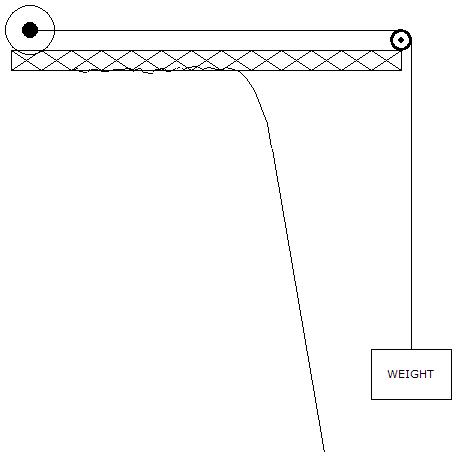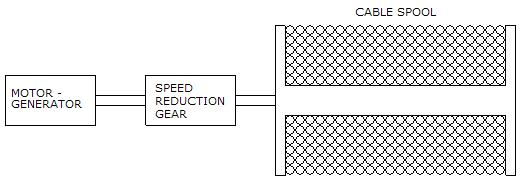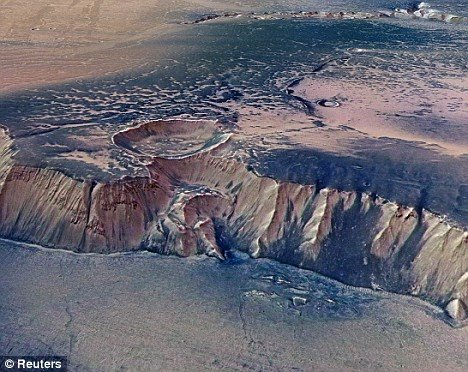HOME Hobby Corner Dave's Circuits Electronic Resources Book Corner Contact Info

 Back of the Envelope Previous Issues

Lifted Weight Energy StorageSome time ago I was reading about all the various ways that off-grid power system owners have tried to store their excess energy.  In a typical off-grid system, a mixture of energy producing devices might all be used at the same time. These may include photovoltaic solar panels and water heating panels mounted on a roof and a few wind generators here and there.
What off-grid users would love to have is a cheap way to capture and store the excess energy produced by their wind generators during a long stretch of windy days.  One storage method, which has been suggested, is a simple “lifted weight” technique.
As illustrated below, a heavy weight is tied to a mechanical system of pulleys and to an efficient speed reduction gear transmission system.  The moving weight is thus converted to the spinning motion of a motor-generator.  To lift the weight, electrical power is routed to the motor.  By reversing the process, energy stored in the weight’s position can then be extracted by spinning the motor in the reverse direction, producing electricity as the weight drops. Let’s see how much energy could be stored using this method.The basic equation used to define the energy stored in a weight is shown below. A block weighing X kilograms lifted to a high of h meters will represent the stored energy in joules, which is also watt-seconds. The 9.8 factor is the gravitational constant for earth. We will make it easier by ignoring all the mechanical losses.

Energy in joules = (weight kg)(9.8)(h meters)

Joules/3600 = watt-hours

OK, let’s say we had a big block of concrete weighing 10,000 kilograms (22,000 pounds).  Let’s say we lifted the weight 10 meters off the ground.  According to the equation, the energy stored is:

10,000kg x 9.8 x 10m = 980,000 joules or 272 watt-hours.

Well, this is not much energy. By comparison, a 12v lead acid car battery with a 50 Amp-hour rating would store 600 watt-hours, which is about twice as much energy as the big lifted weight.  Clearly to be of any use, the concrete block would have to be much larger or the distance the weight moves has to be much longer.  It certainly might be possible use a heavy truss to suspend the weight over a deep canyon but few off-grid homes have such canyons or hills in their back yard.
Could the weight be bigger?  A cubic yard of concrete might weigh about 3800 pounds.  A single big cement truck hauling 10 cubic yards would be able to produce a concrete block weighing perhaps 38,000 pounds or 17,000 kilograms.  A concrete tub like structure could built and then filled with loose rocks or gravel.  So, perhaps a mass weighing 200,000 kilograms could be made and lifted 10 meters of the ground. But, even with this massive block, the total energy stored would only be 5444 watt-hours.  This would be equal to a 12v battery with a 450 Amp-hour rating. This just does not seem very practical.  A larger storage battery would seem like a better solution.
But, suppose this whole concept was moved to Mars. The gravity constant on Mars is only 3.69, so more mass would be needed.  But, Mars has some of the deepest canyons in the solar system. Some canyons measure 8000 meters deep.  Now, if we suspended a weight of 10,000 kilograms on Mars with a height of 8000 meters, the stored energy would be:

10,000kg x 3.69 x 8,000 or 295M joules or 82,000 watt-hours.  Now this is much better.  A 10KW solar panel on Mars, cranking out power for 8 hours would be able lift that heavy weight the whole 8KM distance.  The stored energy could then be used a night.

I think the concept could be scaled way up.  I can imagine using some ultra strong rope (perhaps made of carbon fiber), rated at perhaps 500,000 pounds, which could support a block weighing perhaps 250,000 pounds or 114,000kg.  If such a weight could be moved 8000 meters, then the energy storage would be 932,000 watt-hours or 932 kilowatt-hours.  That is not bad for a simple mechanical energy storage system.Canyons on Mars Canyons on Mars

 July 2010     Issue 11 Page 1 Back Envelope Circuit Diagnosis Experimenter's Corner Good Idea gone Badly New Products Rants & Raves What the World needs Now Wily Widget